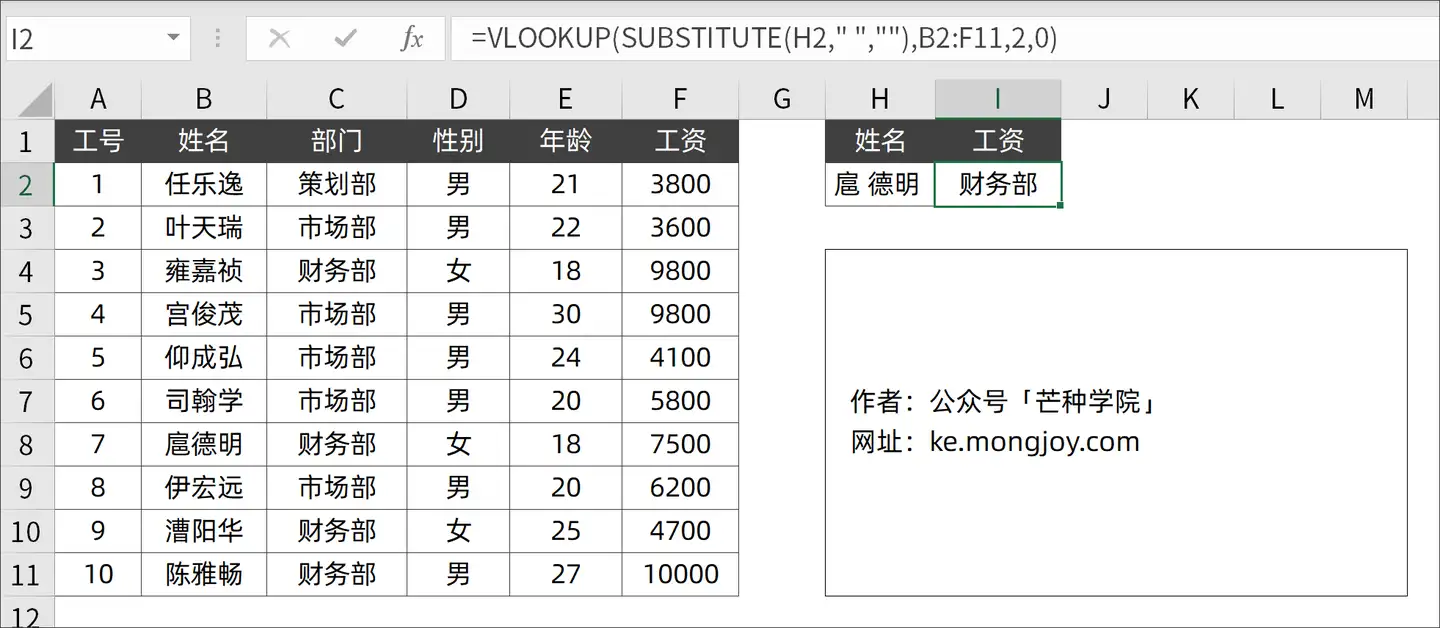# Excel办公技巧-怎样快速掌握Vlookup？

2021-02-05 06:42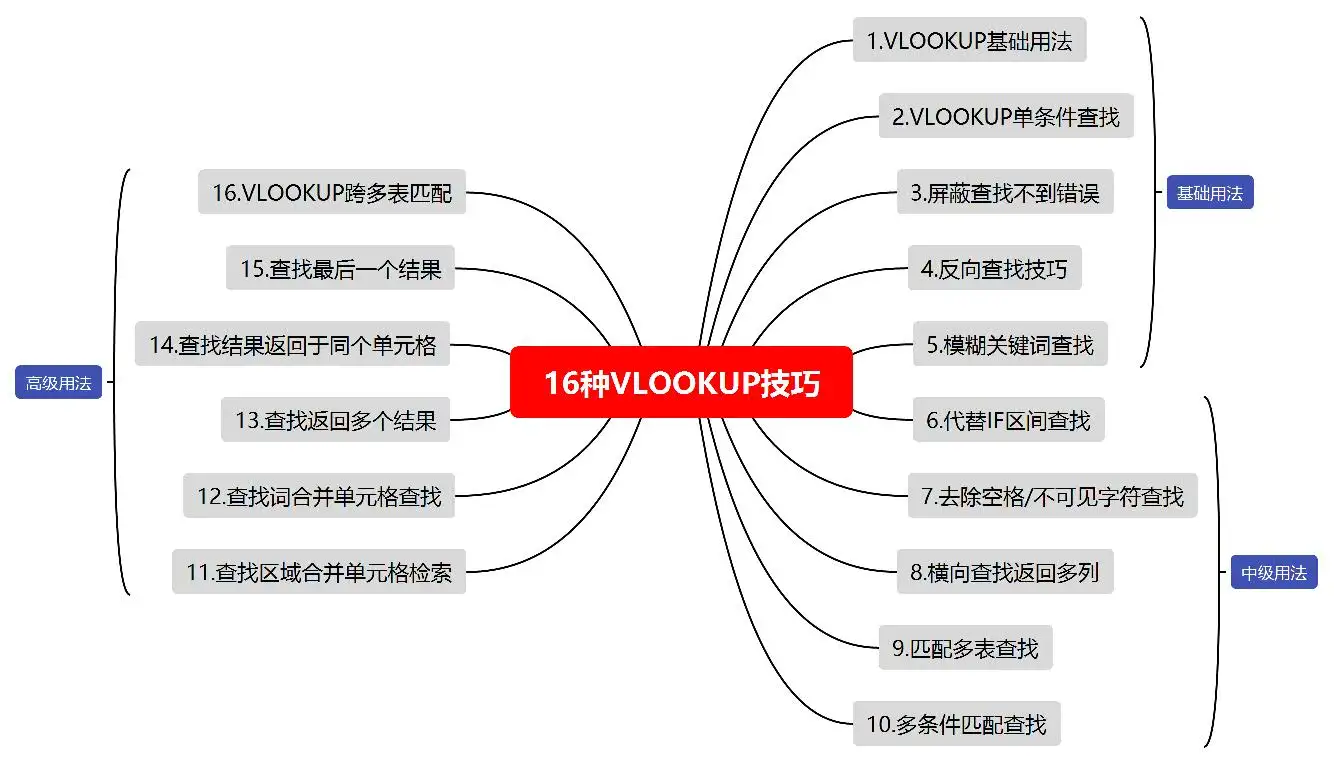## 01. VLOOKUP基础用法

``` = VLOOKUP(查找的值, 查找区域, 返回值所在列数, 匹配模式) ```

• 查找的值：要查找的词或者单元格引用，支持通配符；
• 查找区域：包含查找字段和返回字段的单元格区域，其中查找区域必须位于第 1 列；
• 返回值所在列数：返回值在查找区域中的列数；
• 匹配模式：默认为 1 代表模糊匹配，0代表精确匹配；

## 02. VLOOKUP实现单条件查询

``` =VLOOKUP(H2,A2:F11,6,0) ```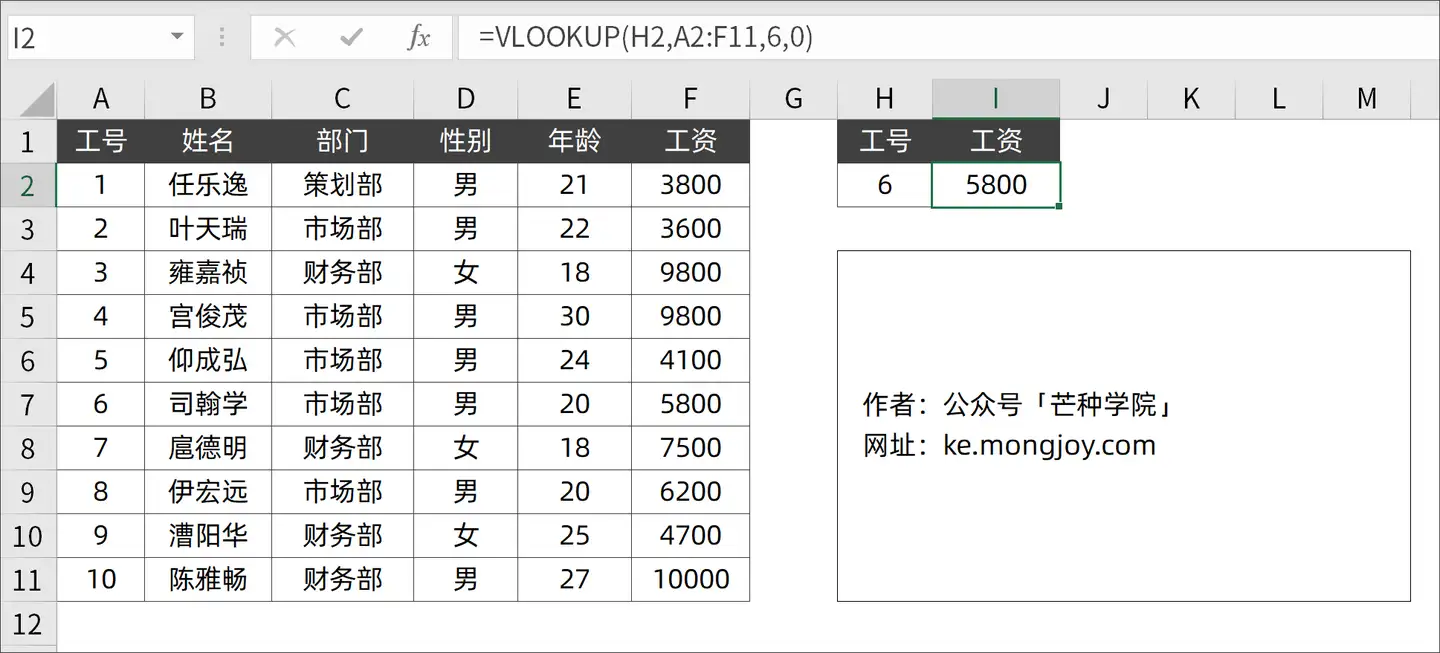1. H2：要查找的单元格引用；
2. A2:F11：要查找的区域；
3. 6：代表「工资」列位于查找区域的第 6 列；
4. 0：代表使用精确匹配模式；

## 03. VLOOKUP屏蔽错误

`=IFERROR(VLOOKUP(H2,A2:F11,6,0),"/")`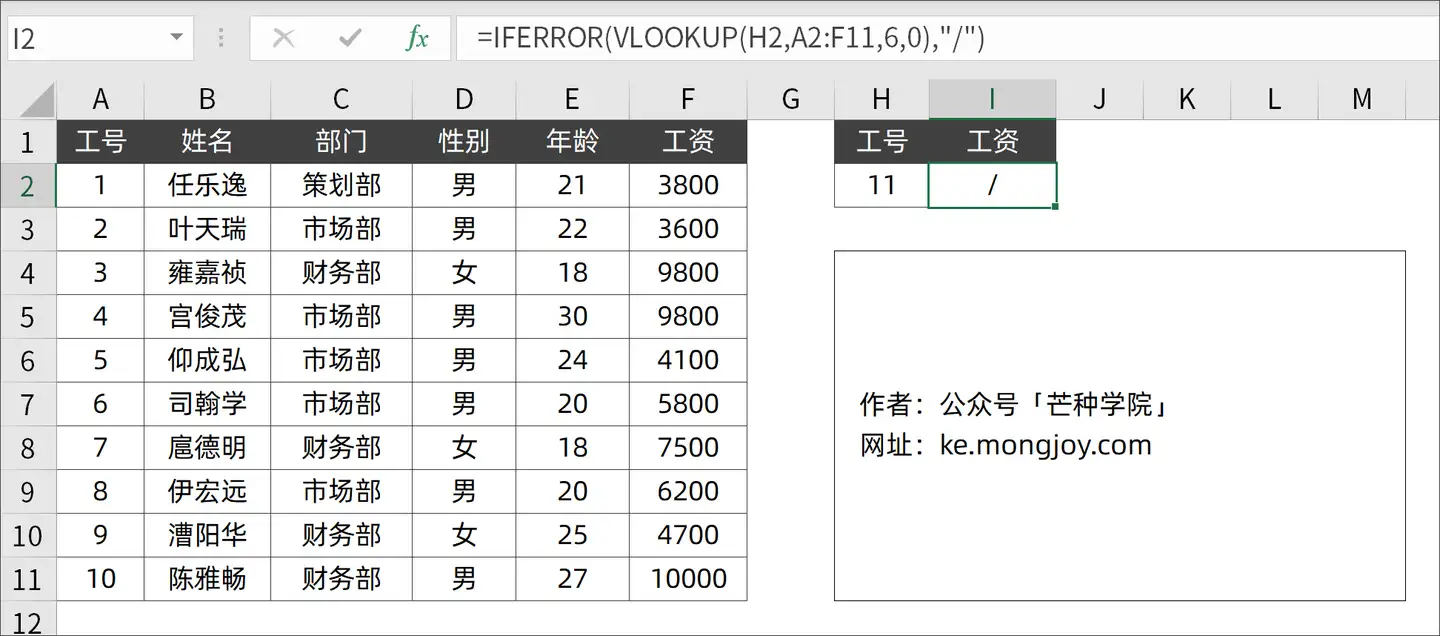IFERROR除了适用于VLOOKUP，在Excel中可以和任何函数进行搭配进行错误屏蔽。

## 04. VLOOKUP实现反向匹配

`=VLOOKUP(H2,IF({1,0},B2:B11,A2:A11),2,0`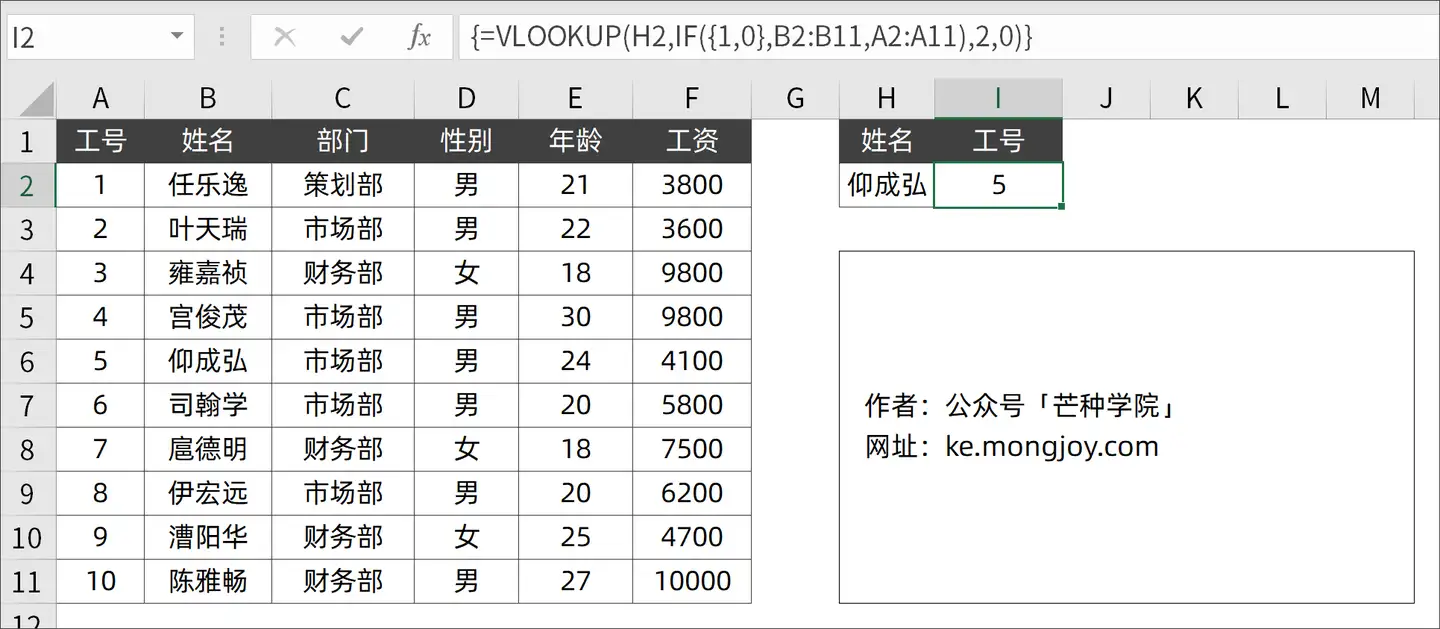## 05. VLOOKUP实现模糊匹配

`=VLOOKUP("*"&H2&"*",B2:F11,5,0)`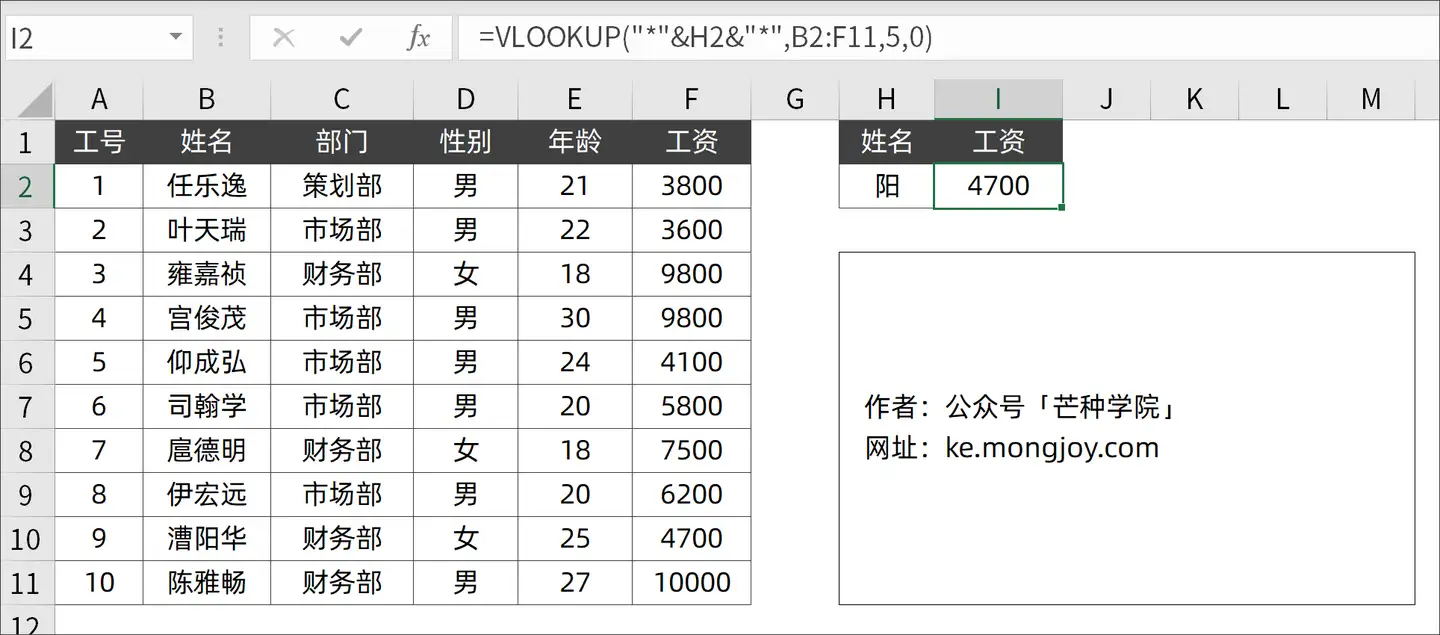## 06. VLOOKUP代替IF多区间匹配

`=VLOOKUP(D2,A2:B6,2,1)`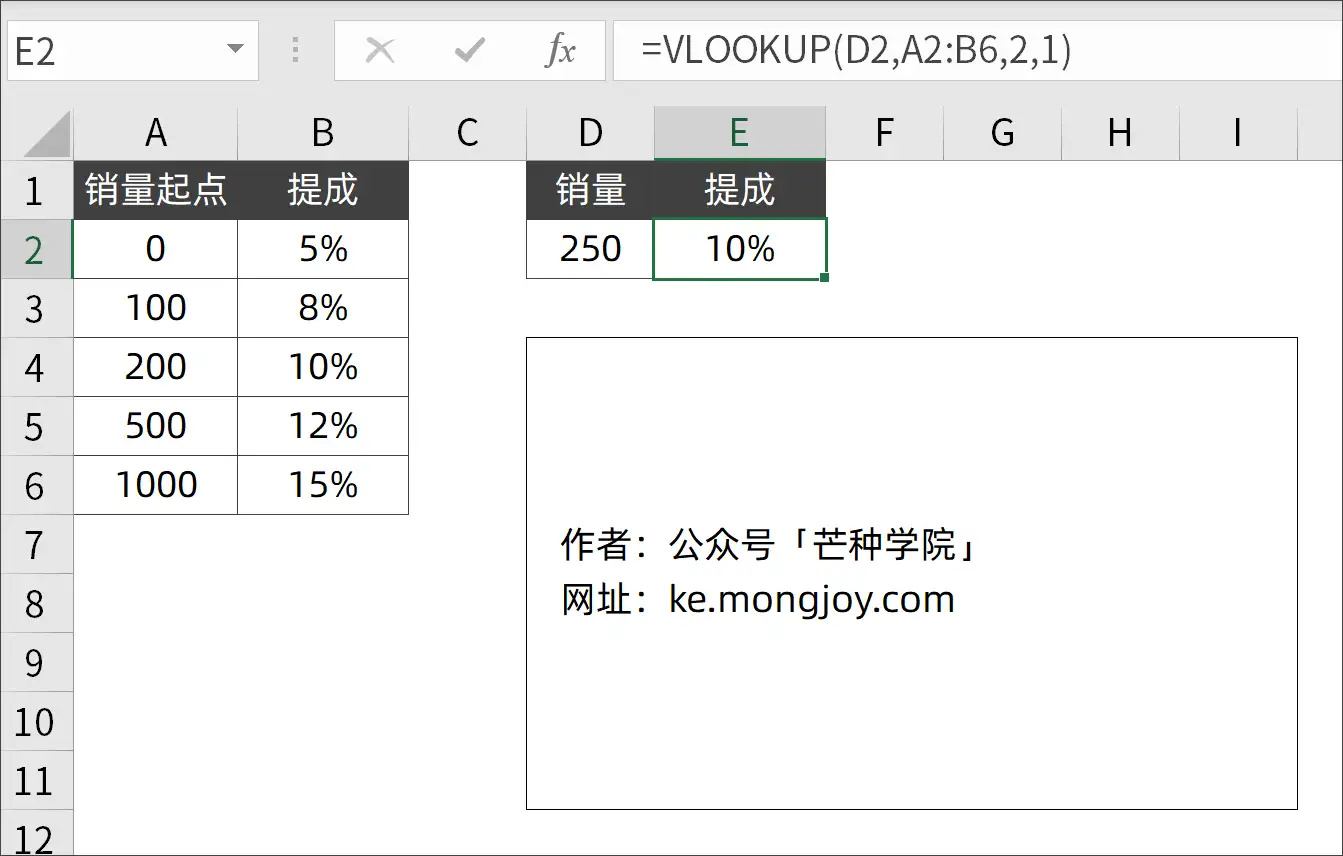## 07. 去除空格/不可见字符匹配

`=VLOOKUP(SUBSTITUTE(H2," ",""),B2:F11,2,0)`

1.SUBSTITUTE 可以替换可见的空格，对于一些不可见字符无能为力；

2.CLEAN 则可以将字符串中的不可见字符通通删除，例如“打印符号/音字符”等；